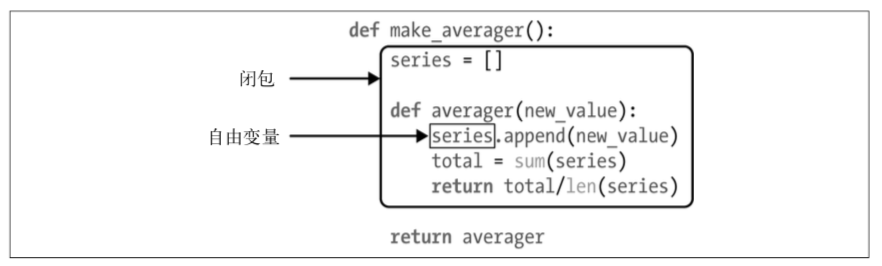# 函数装饰器和闭包

## 装饰器基础知识

```@decorate
def target():
print('running target()')
```

```def target():
print('running target()')
target = decorate(target)
```

## 闭包

```def make_averager():
series = []

def averager(new_value):
series.append(new_value)
total = sum(series)

return averager
```

```>>> avg = make_averager()
>>> avg(10)
10.0
>>> avg(11)
10.5
>>> avg(12)
11.0
```

`averager`函数中，`series`是自由变量(free variable)。这是一个技术术语，指未在本地 作用域中绑定的变量。如下图：averager 的闭包延伸到那个函数的作用域之外，包含自由变量 series 的绑定

```>>> avg.__code__.co_varnames
('new_value', 'total')
>>> avg.__code__.co_freevars
('series',)
```

`series`的绑定在返回的`avg`函数的`__closure__`属性中。`avg.__closure__`中的各个元素对应于`avg.__code__.co_freevars`中的一个名称。这些元素是`cell` 对象，有个`cell_ contents`属性，保存着真正的值。这些属性的值如下所示。

```>>> avg.__code__.co_freevars
('series',)
>>> avg.__closure__
(<cell at 0x10b30ab58: list object at 0x10b525348>,)
>>> avg.__closure__.cell_contents
[10, 11, 12]
```

## nonlocal声明

```def make_averager():
count = 0
total = 0
def averager(new_value):
count += 1
total += new_value

return averager
```

```def make_averager():
count = 0
total = 0

def averager(new_value):
nonlocal count, total
count += 1
total += new_value

return averager
```

## 参数化装饰器

```registry = set()
def register(active=True):
def decorate(func):
print('running register(active=%s)->decorate(%s)'
% (active, func))
if active:
else:
return func

return decorate

@register(active=False)
def f1():
print('running f1()')

@register()
def f2():
print('running f2()')

def f3():
print('running f3()')
```

```def use_logging(level):
def decorator(func):
def wrapper(*args, **kwargs):
if level == "warn":
logging.warn("%s is running" % func.__name__)
elif level == "info":
logging.info("%s is running" % func.__name__)
return func(*args)
return wrapper

return decorator

@use_logging(level="warn")
def foo(name='foo'):
print("i am %s" % name)

foo()
```